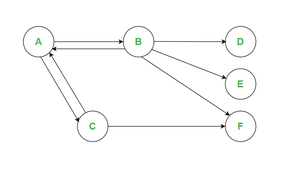GFG App
Open AppBrowser
Continue

# Page Rank Algorithm in Data Mining

Prerequisite: What is Page Rank Algorithm

The page rank algorithm is applicable to web pages. The page rank algorithm is used by Google Search to rank many websites in their search engine results. The page rank algorithm was named after Larry Page, one of the founders of Google. We can say that the page rank algorithm is a way of measuring the importance of website pages. A web page basically is a directed graph which is having two components namely Nodes and Connections. The pages are nodes and hyperlinks are connections.

Let us see how to solve Page Rank Algorithm. Compute page rank at every node at the end of the second iteration. use teleportation factor = 0.8So the formula is,

`PR(A) = (1-β) + β * [PR(B) / Cout(B) + PR(C) / Cout(C)+ ...... + PR(N) / Cout(N)]  `

HERE, β is teleportation factor i.e. 0.8

`NOTE: we need to solve atleast till 2 iteration max.`

Let us create a table of the 0th Iteration, 1st Iteration, and 2nd Iteration.

Iteration 0:

For iteration 0 assume that each page is having page rank = 1/Total no. of nodes

Therefore, PR(A) = PR(B) = PR(C) = PR(D) = PR(E) = PR(F) = 1/6 = 0.16

Iteration 1:

By using the above-mentioned formula

```PR(A) = (1-0.8) + 0.8 * PR(B)/4 + PR(C)/2
= (1-0.8) + 0.8 * 0.16/4 + 0.16/2
= 0.3 ```

So, what have we done here is for node A we will see how many incoming signals are there so here we have PR(B) and PR(C). And for each of the incoming signals, we will see the outgoing signals from that particular incoming signal i.e. for PR(B) we have 4 outgoing signals and for PR(C) we have 2 outgoing signals. The same procedure will be applicable for the remaining nodes and iterations.

NOTE: USE THE UPDATED PAGE RANK FOR FURTHER CALCULATIONS.

```PR(B) = (1-0.8) + 0.8 * PR(A)/2
= (1-0.8) + 0.8 * 0.3/2
= 0.32
PR(C) = (1-0.8) + 0.8 * PR(A)/2
= (1-0.8) + 0.8 * 0.3/2
= 0.32
PR(D) = (1-0.8) + 0.8 * PR(B)/4
= (1-0.8) + 0.8 * 0.32/4
= 0.264
PR(E) = (1-0.8) + 0.8 * PR(B)/4
= (1-0.8) + 0.8 * 0.32/4
= 0.264
PR(F) = (1-0.8) + 0.8 * PR(B)/4 + PR(C)/2
= (1-0.8) + 0.8 * (0.32/4) + (0.32/2)
= 0.392```

This was for iteration 1, now let us calculate iteration 2.

Iteration 2:

By using the above-mentioned formula

```PR(A) = (1-0.8) + 0.8 * PR(B)/4 + PR(C)/2
= (1-0.8) + 0.8 * (0.32/4) + (0.32/2)
= 0.392```

NOTE: USE THE UPDATED PAGE RANK FOR FURTHER CALCULATIONS.

```PR(B) = (1-0.8) + 0.8 * PR(A)/2
= (1-0.8) + 0.8 * 0.392/2
= 0.3568
PR(C) = (1-0.8) + 0.8 * PR(A)/2
= (1-0.8) + 0.8 * 0.392/2
= 0.3568
PR(D) = (1-0.8) + 0.8 * PR(B)/4
= (1-0.8) + 0.8 * 0.3568/4
= 0.2714
PR(E) = (1-0.8) + 0.8 * PR(B)/4
= (1-0.8) + 0.8 * 0.3568/4
= 0.2714
PR(F) = (1-0.8) + 0.8 * PR(B)/4 + PR(C)/2
= (1-0.8) + 0.8 * (0.3568/4) + (0.3568/2)
= 0.4141```

So, the final PAGE RANK for the above-given question is,

My Personal Notes arrow_drop_up